The LIFETEST Procedure
 STRATA Statement

STRATA variable <(list)> <...variable < (list)> > </options> ;
The STRATA statement identifies the variables that determine the strata levels. Strata are formed according to the nonmissing values of these variables. The MISSING option can be used to allow missing values as a valid stratum level. Other options enable you to specify various k-sample tests, stratified tests, or trend tests and to make multiple-comparison adjustments for paired differences.

In the preceding syntax, variable is a variable whose values determine the stratum levels, and list is a list of endpoints for a numeric variable. The values for variable can be formatted or unformatted. If the variable is a character variable, or if the variable is numeric and no list appears, then the strata are defined by the unique values of the strata variable. More than one variable can be specified in the STRATA statement, and each numeric variable can be followed by a list. Each interval contains its lower endpoint but not its upper endpoint. The corresponding strata are formed by the combination of levels. If a variable is numeric and is followed by a list, then the levels for that variable correspond to the intervals defined by the list. The initial interval is assumed to start at, and the final interval is assumed to end at.

The specification of STRATA variables can have any of the following forms:

 list separated by blanks strata age(5 10 20 30) list separated by commas strata age(5,10,20,30) x to y strata age(5 to 10) x to y by z strata age(5 to 30 by 10) combination of the above strata age(5,10 to 50 by 10)

For example, the specification

```   strata Age(5,20 to 50 by 10) Sex;
```

indicates the following levels for the Age variable:This statement also specifies that the Age strata be further subdivided by values of the variable Sex. In this example, there are 6 age groups by 2 sex groups, forming a total of 12 strata.

The specification of several strata variables, such as

```   strata A B C;
```

is equivalent to the A*B*C syntax of the TABLES statement in the FREQ procedure. The number of strata levels usually grows very rapidly with the number of STRATA variables, so you must be cautious when specifying the list of STRATA variables.

When comparing more than two survival curves, a k-sample test tells you whether the curves are significantly different from each other, but it does not identify which pairs of curves are different. A multiple-comparison adjustment of the p-values for the paired comparisons retains the same overall false positives as the k-sample test. Two types of paired comparisons can be made: comparisons between all pairs of curves and comparisons between a control curve and all other curves. You use the DIFF= option to specify the comparison type, and you use the ADJUST= option to select a method of multiple-comparison adjustments.

Table 49.2 summaries the options available in the STRATA statement.

Table 49.2 Options Available in the STRATA Statement

Option

Description

Homogeneity Tests

specifies the group variable for stratified tests

suppresses printing the test statistic and covariance matrix

suppresses any tests

specifies tests corresponding to various weight functions

requests a trend test

Multiple Comparisons

requests a multiple-comparison adjustment

specifies the type of differences to consider

Missing Strata Value

allows missing values as valid stratum values

Options in the STRATA statement are specified after a slash ("/"). The following list describes these options.

specifies the multiple-comparison method for adjusting the p-values of the paired tests. See the section Multiple-Comparison Adjustments for mathematical details; also see Westfall et al. (1999). The adjustment methods include the following:
BONFERRONI
BON

applies the Bonferroni correction to the raw p-values.

DUNNETT

performs Dunnett’s two-tailed comparisons of the control group with all other groups. PROC LIFETEST uses the factor-analytic covariance approximation described in Hsu (1992) and identifies the adjustment in the results as "Dunnett-Hsu." Note that ADJUST=DUNNETT is incompatible with DIFF=ALL.

SCHEFFE

performs Scheffé’s multiple-comparison adjustment.

SIDAK

applies theidák correction to the raw p-values.

SMM
GTE

performs the paired comparisons based on the studentized maximum modulus test.

TUKEY

performs the paired comparisons based on Tukey’s studentized range test. PROC LIFETEST uses the approximation described in Kramer (1956) and identifies the adjustment as "Tukey-Kramer" in the results. Note that ADJUST=TUKEY is incompatible with DIFF=CONTROL.

SIMULATE <(simulate-options)>

computes the adjusted p-values from the simulated distribution of the maximum or maximum absolute value of a multivariate normal random vector. The simulation estimates, the true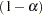th quantile, whereis the value of the ALPHA= simulate-option.

The number of samples for the SIMULATE adjustment is set so that the tail area for the simulatedis within a certain accuracy radiusof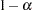with an accuracy confidence of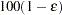%. In equation form,whereis the simulatedand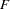is the true distribution function of the maximum; see Edwards and Berry (1987) for details. By default,= 0.005 and= 0.01 so that the tail area ofis within 0.005 of 0.95 with 99% confidence.

The simulate-options include the following:

ACC=value

specifies the target accuracy radiusof a% confidence interval for the true probability content of the estimatedth quantile. The default value is ACC=0.005.

ALPHA=value

specifies the valuefor estimating theth quantile. The default value is the ALPHA= value in the PROC LIFETEST statement, or 0.05 if that option is not specified.

EPS=value

specifies the valuefor a% confidence interval for the true probability content of the estimatedth quantile. The default value for the accuracy confidence is 99%, corresponding to EPS=0.01.

NSAMP=n

specifies the sample size for the simulation. By default,is set based on the values of the target accuracy radiusand accuracy confidence% for an interval for the true probability content of the estimatedth quantile. With the default values for,, and(0.005, 0.01, and 0.05, respectively), NSAMP=12604 by default.

REPORT

specifies that a report on the simulation should be displayed, including a listing of the parameters, such as,, and, as well as an analysis of various methods for estimating or approximating the quantile.

SEED=number

specifies an integer used to start the pseudo-random number generator for the simulation. If you do not specify a seed, or if you specify a value less than or equal to zero, the seed is by default generated from reading the time of day from the computer’s clock.

DIFF=ALL | CONTROL<(’string’ <..., ’string’>)>
specifies which pairs of survival curves are considered for the multiple comparisons.
DIFF=ALL

requests all paired comparisons

DIFF=CONTROL <(’string’ <...’string’>)>

requests comparisons of the control curve with all other curves. To specify the control curve, you specify the quotes strings of formatted values that represent the curve in parentheses. For example, if Cell=’large’ identifies the control group, you specify

```      DIFF=CONTROL('large')
```

If more than one variable is used to identify the curves (for example, if Cell=’large’ and Sex=’F’ represent the control), you specify

```      DIFF=CONTROL('large' 'F')
```

The order of the quoted strings should correspond to the order of the stratum variables. If no specific curve is specified as the control, the first stratum or group value is used.

By default, DIFF=ALL unless you specify ADJUST=DUNNETT, in which case DIFF=CONTROL.
GROUP=variable

specifies the variable whose formatted values identify the various samples whose underlying survival curves are to be compared. The tests are stratified on the levels of the STRATA variables. For instance, in a multicenter trial in which two forms of therapy are to be compared, you specify the variable identifying therapies as the GROUP= variable and the variable identifying centers as the STRATA variable, in order to perform a stratified test to compare the therapies while controlling the effect of the centers.

MISSING

allows missing values to be a stratum level or a valid value of the GROUP= variable.

NODETAIL

suppresses the display of the rank statistics and the corresponding covariance matrices for various strata. If the TREND option is specified, the display of the scores for computing the trend tests is suppressed.

NOTEST

suppresses the k-sample tests, stratified tests, and trend tests.

TREND

computes the trend tests for testing the null hypothesis that thepopulation hazards rate are the same versus an ordered alternatives. If there is only one STRATA variable and the variable is numeric, the unformatted values of the variable are used as the scores; otherwise, the scores are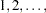in the given order of the strata.

TEST=test-request
TEST=(test-request <...test-request> )

controls the tests produced. Each test corresponds to a different weight function (see the section Nonparametric Tests for the weight functions). The test requests include the following:

ALL

specifies all the nonparametric tests with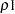=1 and=0 for the Fleming and Harrington test—FLEMING(1,0).

FLEMING(,)

specifies the family of tests in Harrington and Fleming (1982), whereandare nonnegative numbers. FLEMING(,) reduces to the Fleming-Harrington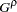family (Fleming and Harrington; 1981) when=0, which you can specify as FLEMING() with one argument. When=0, the test becomes the log-rank test. When=1, the test should be very close to the Peto-Peto test.

LOGRANK

specifies the log-rank test.

NONE

suppresses all comparison tests. Specifying TEST=NONE is equivalent to specify NOTEST.

LR

specifies the likelihood ratio test based on the exponential model.

MODPETO

specifies the modified Peto-Peto test.

PETO

specifies the Peto-Peto test. The test is also referred to as the Peto-Peto-Prentice test.

WILCOXON

specifies the Wilcoxon test. The test is also referred to as the Gehan test or the Breslow test.

TARONE

specifies the Tarone-Ware test.

By default, TEST=(LOGRANK WILCOXON LR) for the k-sample tests, and TEST=(LOGRANK WILCOXON) for stratified and trend tests.Previous Page | Next Page | Top of Page